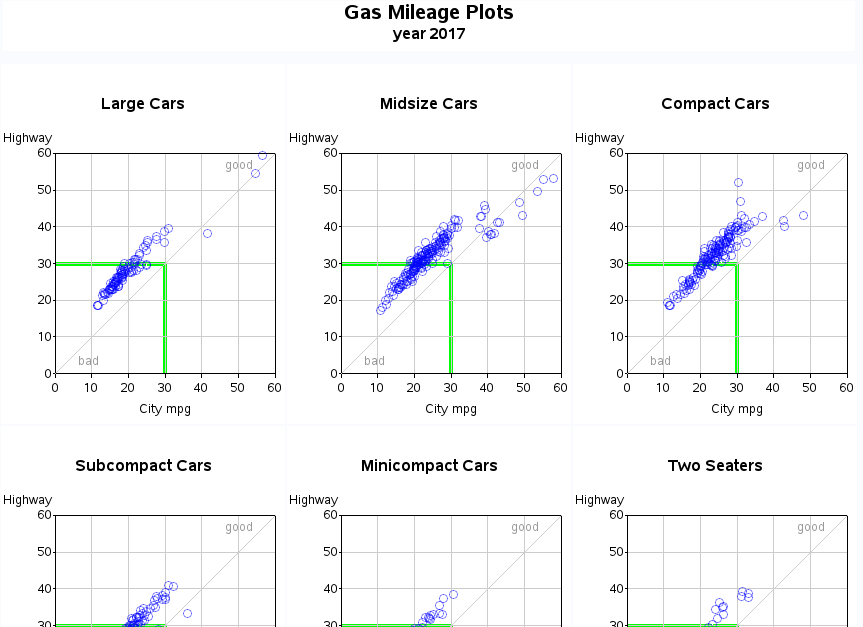## gplot and panel

Hello,

I am trying to do the following I have a dataset with different clustypes. Currently I am plotting each type separately

I would like to do the following

1. Have the charts arranged in a panel with the type in the header of each panel. Main title in the top

2. Output charts in a pdf file  - the  panel first and then followed by each individual chart

Here is a sample data; this shows only two clustering algorithms but I could have 10 or so so I would like to have some flexibility in setting up the panel. I am not sure how to do this any help is appreciated!!

Thanks!

data clusters;

input clustype\$ code\$ id rsqw rsqa size;

datalines;

median a 1 0.8 0.4  1

median b 1 0.85 0.2 1

median c 2 0.4 0.1  2

median d 3 0.9  0.2 2

single a 1 0.8 0.4  1

single b 1 0.85 0.2 1

single c 2 0.4 0.1  2

single d 3 0.9  0.2 2

;

run;

%let clustype = single;

data filter;

set clusters;

if clustype = "&clustype";

run;

data anno_bubbles; set filter;

length function color \$8;

xsys='2'; ysys='2'; hsys='3'; when='b';

x=rsqw; y=rsqa;

function='pie'; rotate=360;

size=size;

if id = 1

then color='#5F9F9F';

if id = 2

then color='#856363';

if id =3  then color='#CD7F32';

if id =4  then color='#9F9F5F';

if id =5  then color='#5F9F9F';

if id =7  then color='#B5A642';

if id =8  then color='#B87333';

if id =9  then color='#BC8F8F';

if id =10  then color='#4F2F4F';

style='psolid'; output;

style='pempty'; color='grayaa'; output;

function='label'; position='2'; size=1.0; text=code;

style=''; color=''; rotate=.; output;

run;

title1 ls=1.5 "&clustype";

symbol1 value=none interpol=none;

axis1 label=(angle=90 "Rsq Within")

order=(0.1 to 1 by 0.05) minor=none offset=(5,5);

axis2 label=("Rsq Across")

order=(0.1 to 1 by .05) minor=none offset=(5,5);

proc gplot data=filter anno=anno_bubbles;

plot rsqw*rsqa /

vaxis=axis1 haxis=axis2;

run;

## Re: gplot and panel

I'm not sure how to do it in pdf output, but if html output would be ok then you could use htmlpanel'ing.

Here's an example: